Not an official ACM page
[Problem H | 1997 ACM Finals problem set | My ACM problem archive | my home page]

# Programming Contest FinalsProblem G

Data in spreadsheets are stored in cells, which are organized in rows (r) and columns (c). Some operations on spreadsheets can be applied to single cells (r,c), while others can be applied to entire rows or columns. Typical cell operations include inserting and deleting rows or columns and exchanging cell contents.

Some spreadsheets allow users to mark collections of rows or columns for deletion, so the entire collection can be deleted at once. Some (unusual) spreadsheets allow users to mark collections of rows or columns for insertions too. Issuing an insertion command results in new rows or columns being inserted before each of the marked rows or columns. Suppose, for example, the user marks rows 1 and 5 of the spreadsheet on the left for deletion. The spreadsheet then shrinks to the one on the right.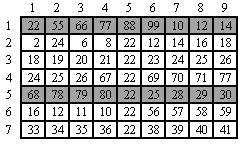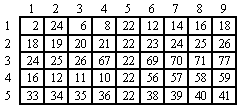If the user subsequently marks columns 3, 6, 7, and 9 for deletion, the spreadsheet shrinks to this.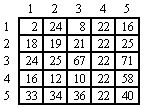If the user marks rows 2, 3 and 5 for insertion, the spreadsheet grows to the one on the left. If the user then marks column 3 for insertion, the spreadsheet grows to the one in the middle. Finally, if the user exchanges the contents of cell (1,2) and cell (6,5), the spreadsheet looks like the one on the right.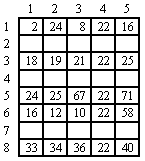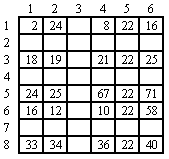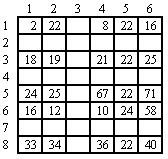You must write tracking software that determines the final location of data in spreadsheets that result from row, column, and exchange operations similar to the ones illustrated here.

### Input and Output

The input consists of a sequence of spreadsheets, operations on those spreadsheets, and queries about them. Each spreadsheet definition begins with a pair of integers specifying its initial number of rows (r) and columns (c), followed by an integer specifying the number (n) of spreadsheet operations. Row and column labeling begins with 1. The maximum number of rows or columns of each spreadsheet is limited to 50. The following n lines specify the desired operations.

An operation to exchange the contents of cell (r1, c1) with the contents of cell (r2, c2) is given by:

``` EX r1 c1 r2 c2 ```
The four insert and delete commands--DC (delete columns), DR (delete rows), IC (insert columns), and IR (insert rows)--are given by:
<command> A x1 x2 ... xA
where <command> is one of the four commands; A is a positive integer less than 10, and x1, ... xA are the labels of the columns or rows to be deleted or inserted before. For each insert and delete command, the order of the rows or columns in the command has no significance. Within a single delete or insert command, labels will be unique.

The operations are followed by an integer which is the number of queries for the spreadsheet. Each query consists of positive integers r and c, representing the row and column number of a cell in the original spreadsheet. For each query, your program must determine the current location of the data that was originally in cell (r,c). The end of input is indicated by a row consisting of a pair of zeros for the spreadsheet dimensions.

For each spreadsheet, your program must output its sequence number (starting at 1). For each query, your program must output the original cell location followed by the final location of the data or the word GONE if the contents of the original cell location were destroyed as a result of the operations. Separate output from different spreadsheets with a blank line.

The data file will not contain a sequence of commands that will cause the spreadsheet to exceed the maximum size.

```7 9
5
DR   2  1 5
DC  4  3 6 7 9
IC  1  3
IR  2  2 4
EX 1 2 6 5
4
4 8
5 5
7 8
6 5
0 0
```

### Output for the Sample Output

```Spreadsheet #1
Cell data in (4,8) moved to (4,6)
Cell data in (5,5) GONE
Cell data in (7,8) moved to (7,6)
Cell data in (6,5) moved to (1,2)
```